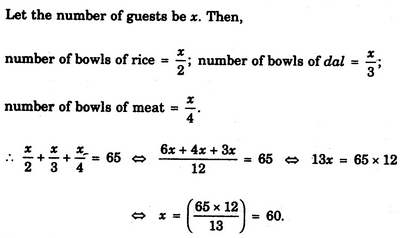# Verbal Reasoning - Arithmetic Reasoning

Exercise : Arithmetic Reasoning - Section 1
46.
The total number of digits used in numbering the pages of a book having 366 pages is
732
990
1098
1305
Explanation:

Total number of digits

= (No. of digits in 1- digit page nos. + No. of digits in 2-digit page nos. + No. of digits in 3- digit page nos.)

= (1 x 9 + 2 x 90 + 3 x 267) = (9 + 180 + 801) = 990.

47.
In a family, each daughter has the same number of brothers as she has sisters and each son has twice as many sisters as he has brothers. How many sons are there in the family ?
2
3
4
5
Explanation:

Let d and s represent the number of daughters and sons respectively.

Then, we have :

d - 1 = s and 2 (s - 1) = d.

Solving these two equations, we get: d = 4, s = 3.

48.
At a dinner party every two guests used a bowl of rice between them, every three guests used a bowl of dal between them and every four used a bowl of meat between them. There were altogether 65 dishes. How many guests were present at the party ?
60
65
90
None of these
Explanation:49.
Ayush was born two years after his father's marriage. His mother is five years younger than his father but 20 years older than Ayush who is 10 years old. At what age did the father get married ?
23 years
25 years
33 years
35 years